Courses

# OP AMP MSQ

## 10 Questions MCQ Test Topic wise Tests for IIT JAM Physics | OP AMP MSQ

Description
This mock test of OP AMP MSQ for Physics helps you for every Physics entrance exam. This contains 10 Multiple Choice Questions for Physics OP AMP MSQ (mcq) to study with solutions a complete question bank. The solved questions answers in this OP AMP MSQ quiz give you a good mix of easy questions and tough questions. Physics students definitely take this OP AMP MSQ exercise for a better result in the exam. You can find other OP AMP MSQ extra questions, long questions & short questions for Physics on EduRev as well by searching above.
*Multiple options can be correct
QUESTION: 1

### Common-mode rejection ratio is given by

Solution: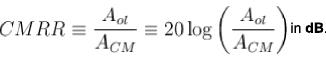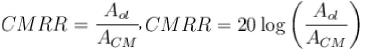*Multiple options can be correct
QUESTION: 2

### If Av(d) = 3500 and ACM = 0.35, then CMRR is :

Solution: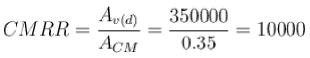Expressed in dB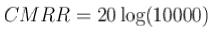= 80 dB
The correct answers are: 10000, 80 dB

*Multiple options can be correct
QUESTION: 3

### If a certain Op-Amp has a closed loop gain of 20 and an upper critical frequency of 10 MHz, the gain bandwidth product is :

Solution:

GBW= 20 x 10 = 200MHz and gain bandwidth product is also defined as the frequency with unity gain.
The correct answers are: 20 MHz, unity gain frequency

*Multiple options can be correct
QUESTION: 4

The Op-Amp has the following parameters; Aol = 50000; Zin = 4MΩ and Zout = 50Ω. The values of input impedance, output impedance and close loop voltage gains are :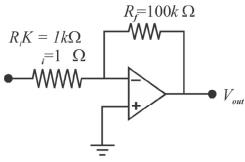Solution: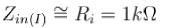The feedback attenuation B is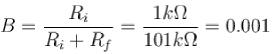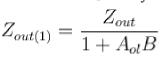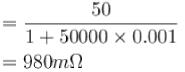zero for all practical purposes. The closed loop voltage gain is.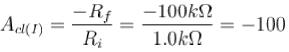The correct answers are: Zout = 980mΩ, Acl(t) = -100

*Multiple options can be correct
QUESTION: 5

The circuit shown in the figure can be used as a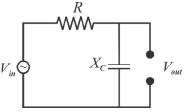Solution:

The given circuit is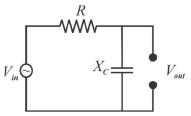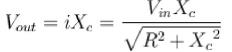It is an integrator and low-pass filter.
The correct answers are: low pass filter, Integrator

*Multiple options can be correct
QUESTION: 6

The inverting input terminal of an operational amplifier (Op-Amp) is shorted with output terminal apart from being grounded. A voltage signal V1 is applied to the noninverting input terminal of the Op-Amp. Under this configuration the Op-Amp function is as :

Solution:

The lowest gain can be obtained from a non-inverting amplifier with feedback 1.
When the non-inverting amplifier is configured for unit gain, it is called a voltage follower because the output voltage is equal to and in phase with the input. In other words, in the voltage follower, the output follows the input. To obtain the voltage follower from non-inverting amplifier of figure 1. simply open R1 and short Rf The resulting circuit is shown is figure 2. In this figure, all the output voltages are feedback into the inverting terminal of the Op-Amp ; consequently the gain of the feedback is 1.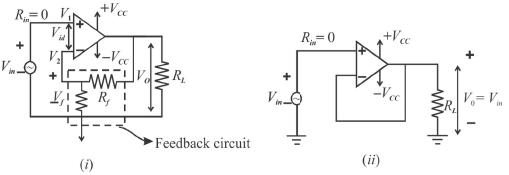The correct answer is: a voltage follower

*Multiple options can be correct
QUESTION: 7

A differential amplifier.

Solution:

Any differential amplifier has two inputs and two outputs (one is Vout and other is ground potential) The correct answers are: is a part of an Op-Amp, has two output

*Multiple options can be correct
QUESTION: 8

The data of Op-Amp shown in figure is.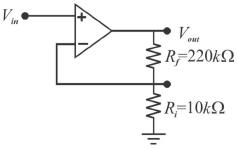Zin = 2MΩ, ZOut = 75Ω and AoI = 200000, then the correct matching pairs is.

Solution:

The attenuation, B, of the feedback circuit is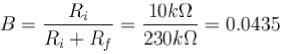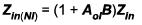= [1 + (200000)(0.0435)](2MΩ)
= 17.4 GΩ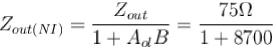= 8.6mΩ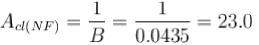The correct answers are: Attenuation of the feedback = +0.0435, Input impedance = +17.4 GΩ

*Multiple options can be correct
QUESTION: 9

In order to obtain a solution of the differential equation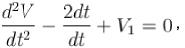involving voltage V(t) and V1 an Op-Amp circuit would require at least.

Solution:

Since, it is a two degree linear differential equation, hence, two integrators are required, also one adder to calculate the sum of outputs.

*Multiple options can be correct
QUESTION: 10

A certain Op-Amp has an open loop voltage gain of 1,00,000 and a common mode gain of 0.2. The CMRR is :

Solution:

Aol = 100000
Acm = 0.2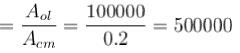Expressed in decibels
CMRR =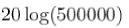= 114dB
The correct answers are: 500000, 114 dB